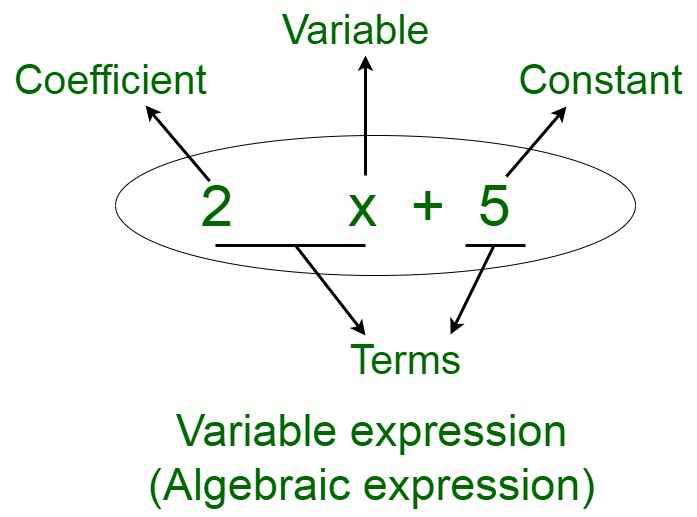# Simplify 7x2(3x – 9) + 3 and find its values for x = 4 and x = 6

• Last Updated : 13 Oct, 2021

An algebraic expression, which is also known as a variable expression is an equation composed of variable terms formed from the combination of constants and variables. These components are joined together using operations, like addition, subtraction, multiplication, or division. The constants accompanied by the variable in each term are referred to as the coefficient.### Solve the equation 7x2(3x – 9) + 3 for x = 4 and x = 6

Solution:

⇒ 7x2(3x – 9) + 3

Find the solution for 7x2(3x – 9)

By using distributive law which states that;

a(b – c) = ab – ac

So according to the law

⇒ (7x2 × 3x)  – (7x2 × 9)

⇒ 21x3 – 63x2

Therefore,

⇒ 7x2(3x – 9) + 3 = 21x3 – 63x2 + 3

Now we have to solve for the equation

21x3 – 63x2 + 3

Further,

For x = 4,

⇒ 21x3 – 63x2 + 3

⇒ 21 × 43 – 63 × 42 + 3

⇒ 1344 – 1008 + 3

⇒ 336 + 3

⇒

Further,

Now for x = 6,

⇒ 21x3 – 63x2

⇒ 21 × 63 – 63 × 62 + 3

⇒ 2268 + 3

⇒ 2271

Therefore,

The algebraic expression ⇒ 7x2(3x – 9) + 3

For the value of x = 4 is 339

For the value of x = 6 is 2271

### Sample Questions

Question 1. By applying suitable algebraic identity, Find 10502

Solution:

By applying the algebraic identity in the question: (a + b)² = a² + 2ab + b²

Thus,

1050 = 1000 + 50

Therefore,

10502 = (1000 + 50)2

Here,

a = 1000

b = 50

(1000 + 50)2 = (1000)² + 2 × 1000 × 50

50)²

= 1000000 + 100000 + 2500

Therefore,

10502 = 1102500.

Question 2. Simplify 82 + 2×(5x – 7). For the values of x = 2 and x = -2?

Solution:

Here we have,

82 + 2 × (5x – 7)

For x = 2

Substitute value of x = 2 in the equation

= 82 + 2 × (5x – 7)

= 82 + (2 × 5x  – 2 × 7)

= 82 + (10x – 14)

= 82 + 10x – 14

= 82 – 14 + 10 × 2

= 82 – 14 + 20

= 88

For x = -2

Substitute value of x = -2 in the equation

= 82 + 2 × (5x – 7)

= 82 + (2 × 5x  R

2 × 7)

= 82 + (10x – 14)

= 82 + 10x – 14

= 82 – 14 + 10 × (-2)

= 82 – 14 – 20

= 48

Question 3. Simplify 24 × 7  + x(365 – 65). For the value of x = 1 and x = -1

Solution:

Here we have

24 × 7 + x(365 – 65)

For x = 1

Substitute value of x = 1 in the equation

= 24 × 7 + x(365 – 65)

= 168 + x(365 – 65)

= 168 + 365x – 65x

= 168 + 300x

= 168 + 300 × 1

= 168 + 300

= 468

For x = -1

Substitute value of x = -1 in the equation

= 24 × 7 + x(365 – 65)

= 168 + x(365 – 65)

= 168 + 365x – 65x

= 168 + 300x

= 168 + 300 × (-1)

= 168 – 300

= -132

Question 4. Subtract the polynomials.

(6x + 3) from (-8x + 6)

And simplify for x = 4

Solution:

(6x + 3) from (-8x + 6)

= (-8x + 6) – (6x + 3)

= -8x + 6 – 6x – 3

= -8x -6x + 6 – 3

= -14x + 3

For x = 4

Substitute value of x = 4 in the equation

= -14 �

sp;4 + 3

= -56 + 3

= -53

Question 5. Solve the equation 5x2(6x – 7) + 5 for x = 2 and x = 4

Solution:

5x2(6x – 7) + 5

Find the solution for

5x2(6x – 7)

By using distributive law which states that;

a(b – c) = ab – ac

So according to the law

⇒ (5x2 × 6x)  – (5x2 × 7)

�� 30x3 – 35x2

Therefore,

⇒ 5x2(6x – 7) + 5 = 30x3 – 35x2 + 5

Now we have to solve for the equation

30x3 – 35x2 + 5

Further,

For x = 4,

⇒ 30x3 – 35x2 + 5

⇒ 30 × 43 – 35 × 42 + 5

⇒ 1920 – 560 + 5

⇒ 1360 + 5

⇒ 1365

Further,

Now for x = 6,

⇒ 30x3 – 35x2 + 5

⇒ 30 × 63 – 35 × 62 + 5

⇒ 6480 – 1260 + 5

⇒ 5220 + 5

⇒ 5225

Therefore,

The algebraic expression ⇒ 5x2(6x – 7) + 5

For the value of x = 4 is 1365

For the value of x = 6 is 5225

My Personal Notes arrow_drop_up